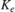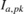# Parameterize a Permanent Magnet Synchronous Motor

This example shows how to estimate the back EMF and torque constants of a blackbox permanent magnet synchronous motor (PMSM) with an unknown flux linkage. You can use either the back EMF or torque constant to describe the flux linkage and parameterize a Simscape™ Electrical™ PMSM block. This parameterization allows you to accurately replicate the blackbox motor's behavior in simulation.

In this example, the number of pole pairs, stator resistance and stator inductances of the simulation and blackbox PMSMs are already specified to match.

### Open Model

Open the model.### Set Back EMF Constant

Select the back EMF parameterization option for the simulation PMSM and initialize its value to an arbitrary default value of 0.1 V*s/rad.

Alternatively, you can set the back EMF parameterization option and specify its value from the PMSM block mask by selecting Specify back EMF under Permanent magnet flux linkage parameterization and specifying Back EMF constant.

### Compare Results

Simulate the model and plot the voltage over time across the a-winding for both the experimental and simulation PMSMs. Observe that there is a significant difference in voltage amplitude between the experimental and simulation PMSMs.### Calculate Voltage Constant

Calculate the voltage constantof the blackbox experimental PMSM by using the defining equation,

whereis the angular velocity of the PMSM shaft, andis the voltage drop across the a-winding of the motor. This equation is valid only when the current through the windings is very small. The small current restriction is enforced in the model by attaching each of the winding free ends to ground via a very high resistance, effectively making each winding an open-circuit.is calculated by taking the average of the maximum and minimum values of the voltage drop across the a-winding, and dividing the result by two. You can improve the accuracy of this calculation by first filtering out the high frequency noise of the measured voltage signal:

The angular velocity can be measured directly from the source block:

The back EMF constant can now be calculated from the above results:

 0.1803 

### Change Back EMF Constant

Change the back EMF constant for the simulation motor to the estimated value, calculated in the previous section.

### Compare New Results

Resimulate the model and plot the voltage across the a-winding. The new parameterization of the voltage constant improves the simulation PMSM's ability to replicate the behavior of the experimental PMSM.### Calculate Torque Constant

Calculate the torque constantof the blackbox PMSM using the defining equation:,

whereis the peak current through the a-winding andis the overall mechanical torque driving the PMSM.

 0.1797As expected, the measured torque and back EMF constants have approximately the same value (0.18) and are related to the permanent magnet flux linkagevia the number of pole pairsof the motor:You can parameterize the permanent magnet flux linkage of the motor by specifying any of,orin the Simulation PMSM block mask. Back EMF and torque constants are more commonly given than permanent magnet flux linkage on motor datasheets.